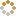﻿ Ningbo University of Technology Online Judge ::  I Have No Time
• ###  I Have No Time

• 时间限制: 1000 ms　内存限制: 65535 K
• 问题描述
• Get up! Get up!!! It's 7:45 now. I'll be late for the class at 8:00.
There're several ways you can get to classroom. But the problem is that there's only little time left.
So please tell me how many Optimized Ways that I can choose?

The Optimized Way means: The minimum time of from next position to the class must be less than the minimum time of from current position to the class.
• 输入
• The first line of this problem is an integer T, means the number of cases.
The first line of each is a integer N (1 <= N <= 20), means the side of the school (You can assume is a square and the dormitory is at (1, 1) while the classroom is at (N, N)).
Then follow an N * N matrix. Each matrix cell (1 <= cell <= 1000) indicates the time you'll spend while you're going through this point.
• 输出
• For each tests, you should output the number of Optimized Ways.
• 样例输入
• ```1
5
1 1 1 1 1
1 1 1 1 1
1 1 1 1 1
1 1 1 1 1
1 1 1 1 1
```
• 样例输出
• ```70
```
• 提示
• `无`
• 来源
• `Ne123123`
• 操作
﻿